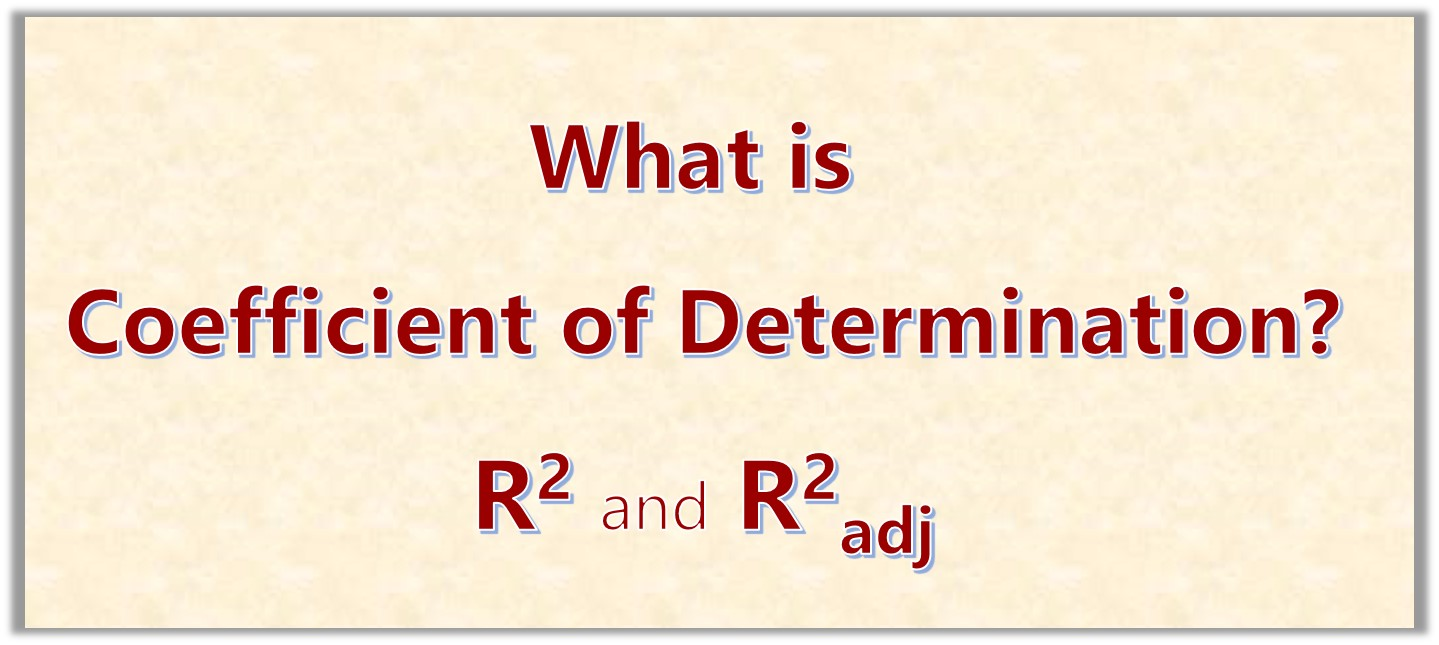# What is Coefficient of Determination in Linear RegressionIf you are learning linear regression, then you need to clearly understand the concept of Coefficient of Determination R2 and the Adjusted Coefficient of Determination R2adj.

I am going to explain these concepts in a very easy way.

We are going to cover the following:

### 1. What is Coefficient of Determination?

The coefficient of determination, is used to determine the proportion of the variation of one of the variables  that is predictable from the other variable.

Look at the table below. What do you think is the relationship between X and Y?
It seems that Y equal X/2. But carefully looking at the table we see that this is not exactly true for two of the data points.

But we can say that 80% of the time, Y is X/2. This means that the coefficient of determination is 0.8 (or 80%)

 Y X 1.0 2.0 2.0 4.0 3.0 7.0 4.0 8.0 5.0 10.0 6.0 12.3 7.0 14.0 8.0 16.0 9.0 18.0 10.0 20.0

Table 1: For 8 out of the 10 points, y=x/2

The coefficient of determination is a measure of how certain we are in making predictions from a certain model.

It determines the ratio of the explained variation to the total variation.

The value of R2 ranges from 0 to 1, that it:
0 < R2 < 1

It denotes the strength of the linear association between x and y. When we are using a line of best fit, then the coefficient of determination represents the percent of the data that is closest to the line of best fit.

For example, if R = 0.89 then R2 = 0.792 which means that 79.2% of the total variation in y can be explained bz the linear relationship between y and x (as described by the regression equation, in our case it is y = x/2.

The other 20.8% of the variation remains unexplained.

So we can say that the coefficient of determination is a measure of how well the regression line represents the data.

Formula for R2 is given by:

### 2. Properties of Coefficient of Determination

Let’s now outline some of the properties of R2  that you need to know. To get used to these properties, take some time to write then out in your note.

0 ≤ R2  ≤  1  if  f(X) = r(X) = E(Y | X)
if X and Y are independent, then R2 = 0
if Y = f(X) then R2 = 1
if f(X) = a*X + b* then the theoretical linear regression is given by R2 = (R(X,Y))2
if the joint distribution of X and Z is normal, then R2 = (R(X,Y))2